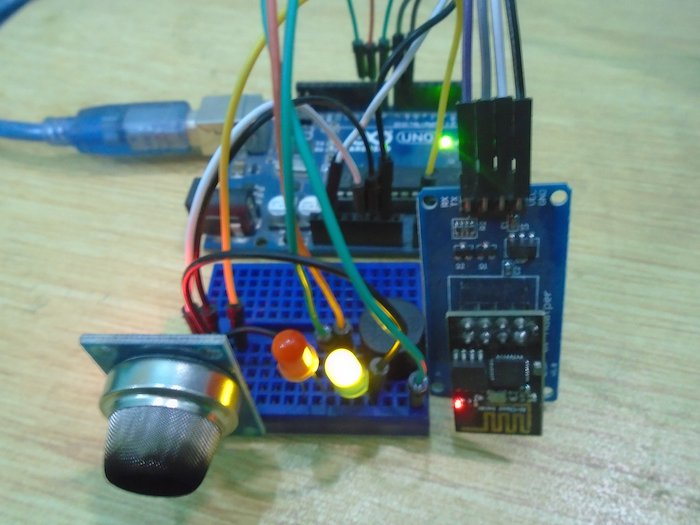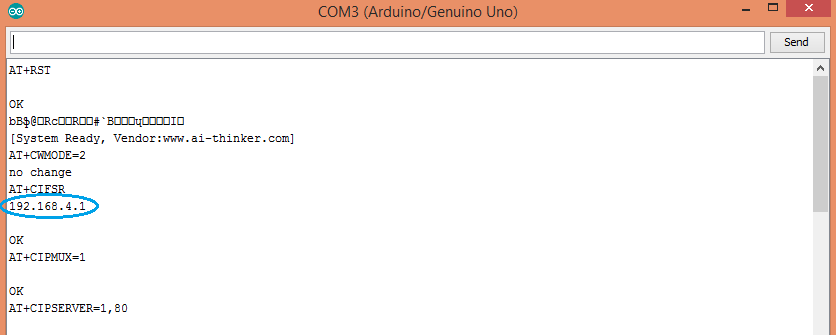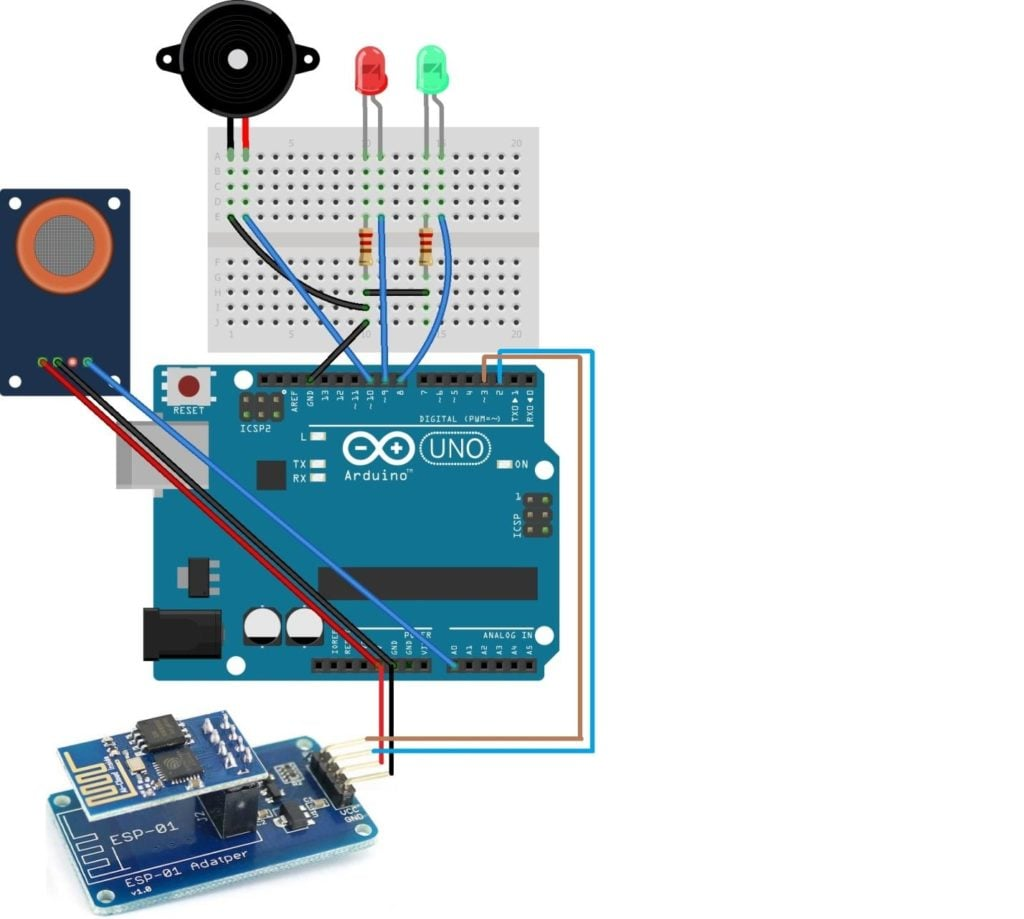Use an Arduino, an ESP8266, and a gas sensor to make an IoT smoke alarm.

In this project, we are going to make an IoT smoke detection system in which the MQ-2 smoke sensor will sense if there is smoke nearby. If smoke is nearby, then the buzzer will start beeping and the red LED will light up and a warning will be displayed on a web page which we will create using the ESP8266 module. This web page will be accessible using any connected device like a mobile, tablet, or PC.### How Does the Smoke Alarm Work?

The MQ-2 smoke sensor will give the output in the form of analog voltage. We have set a condition in our code that if the output value of the sensor is greater than 400, then the buzzer will start to beep and the red LED will light up; and if the output value of the sensor is less than 400, then the buzzer will remain quiet and the green LED will light up.

The ESP used here will create a web page at an IP address and will send the data to this IP address and will print the data there. After uploading the code, this IP address can be seen in the serial monitor as shown below.When you type this IP address in your browser, then the output of the project will be shown there like in the figure below.### Circuit Diagram

First of all, connect the ESP8266 module with the Arduino. To connect the ESP8266 properly with the Arduino, we used an ESP-01 adapter module which will make the connection very easy. This adapter module has a built-in 5V to the 3.3V regulator, which means that you will not have to use any resistors.

Connect the ESP-01 adapter's VCC pin to the 5V pin on the Arduino and the ESP-01 adapter's GND to GND on the Arduino. Next, connect the TX pin from the adapter to the pin 2 on the Arduino and the RX pin from the adapter to the pin 3 on the Arduino.

Then, Connect the MQ-2 gas sensor with the Arduino. Connect the VCC and the GND on the gas sensor to the 5V and GND pins on the Arduino. Then connect the A0 pin on the MQ-2 gas sensor to the A0 on the Arduino.

After that, connect the Buzzer and the LEDs to the Arduino. Connect the positive on the buzzer with pin 10 on the Arduino and the negative on the buzzer with GND on the Arduino. Then connect the negative side of the LEDs to the GND through the 220-ohm resistor and the positive side to pins 8 and 9 on the Arduino.### Code Explanation

First of all, add the software serial library. The software serial library will allow us to use the TX and RX communication on the Arduino's other pins rather than only using the default TX and RX pins. Then we defined that where we have connected these TX and RX pins on the Arduino.

```#include <SoftwareSerial.h>

#define DEBUG true

SoftwareSerial wifi_module(2,3); // Connect TX pin of esp to the pin 2 of Arduino and RX pin of esp to the pin 3 of Arduino```

In the lines below, we have declared the pins for the LEDs, buzzer, and the smoke sensor.

```int red_led_pin = 9;

int green_led_pin = 8;

int buzzer_pin = 10;

int smoke_sensor_pin = A0;```

In the setup function, first, we set the baud rate for the serial communication and for the wifi module at 9600. Then we declared the led pins and the buzzer pin as the output pins because we will give the output from these pins to the LEDs and the buzzer. Last, we declared the smoke sensor pin as the input because the smoke sensor pin will take the input from the sensor and will give it to the Arduino.

```Serial.begin(9600);

wifi_module.begin(9600); // Set the baudrate according to your esp8266

pinMode(red_led_pin, OUTPUT);

pinMode(green_led_pin, OUTPUT);

pinMode(buzzer_pin, OUTPUT);

pinMode(smoke_sensor_pin, INPUT);```

The lines will call the function and will set up a server at an IP address provided by the ESP. The ESP will then send the data at this IP address.

```  esp8266_command("AT+RST\r\n",2000,DEBUG); // reset module

esp8266_command("AT+CWMODE=2\r\n",1000,DEBUG); // configure as access point

esp8266_command("AT+CIPMUX=1\r\n",1000,DEBUG); // configure for multiple connections

esp8266_command("AT+CIPSERVER=1,80\r\n",1000,DEBUG); // turn on server on port 80```

In the loop function, we read the values from the smoke sensor and then applied a condition that if the output value is greater than 400, then the red LED will light and the buzzer will start to beep. If the output value is less than 400, then the green LED will light up and the buzzer will remain quiet.

```int analogSensor = analogRead(smoke_sensor_pin);

if (analogSensor > 400)

{

digitalWrite(red_led_pin, HIGH);

digitalWrite(green_led_pin, LOW);

tone(buzzer_pin, 1000, 200);

}```

The below lines will print the data on the web page. Firstly, we will print the “IoT Smoke detection system” on the top. Then on the second line, we will print the smoke value and on the third line, we will print “Everything Normal” or “DANGER! Move Somewhere Else” depending on the condition.

```String webpage = "<h1>IOT Smoke Detection System</h1>";

webpage +="<p>Smoke Value is ";

webpage += analogSensor;

webpage +="</p>";

if (analogSensor > 400)

{

webpage +="<h5>DANGER! Move Somewhere Else</h5>";

}

else

{

webpage +="<h4>Everything Normal</h4>";

}```

The code below will send the commands to the ESP and will print the output of the ESP on the serial monitor.

```String esp8266_command(String command, const int timeout, boolean debug)

{

String response = "";

wifi_module.print(command);

long int time = millis();

while( (time+timeout) > millis())

{

while(wifi_module.available())

{

response+=c;

}

}```
So that’s it, hope you get it working! Furthermore, you can also check out my blog to see some of my other projects on ElectronicsHobbyist.com.

### Code

```#include <SoftwareSerial.h>

#define DEBUG true

SoftwareSerial wifi_module(2,3); // Connect TX pin of esp to the pin 2 of Arduino and RX pin of esp to the pin 3 of Arduino

int red_led_pin = 9;

int green_led_pin = 8;

int buzzer_pin = 10;

int smoke_sensor_pin = A0;

void setup()

{

Serial.begin(9600);

wifi_module.begin(9600); // Set the baudrate according to your esp8266

pinMode(red_led_pin, OUTPUT);

pinMode(green_led_pin, OUTPUT);

pinMode(buzzer_pin, OUTPUT);

pinMode(smoke_sensor_pin, INPUT);

esp8266_command("AT+RST\r\n",2000,DEBUG); // reset module

esp8266_command("AT+CWMODE=2\r\n",1000,DEBUG); // configure as access point

esp8266_command("AT+CIPMUX=1\r\n",1000,DEBUG); // configure for multiple connections

esp8266_command("AT+CIPSERVER=1,80\r\n",1000,DEBUG); // turn on server on port 80

}

void loop()

{

if (analogSensor > 400)

{

digitalWrite(red_led_pin, HIGH);

digitalWrite(green_led_pin, LOW);

tone(buzzer_pin, 1000, 200);

}

else

{

digitalWrite(red_led_pin, LOW);

digitalWrite(green_led_pin, HIGH);

noTone(buzzer_pin);

}

if(wifi_module.available())

{

if(wifi_module.find("+IPD,"))

{

delay(1000);

String webpage = "<h1>IOT Smoke Detection System</h1>";

webpage +="<p>Smoke Value is ";

webpage += analogSensor;

webpage +="</p>";

if (analogSensor > 400)

{

webpage +="<h5>DANGER! Move Somewhere Else</h5>";

}

else

{

webpage +="<h4>Everything Normal</h4>";

}

String cipSend = "AT+CIPSEND=";

cipSend += connectionId;

cipSend += ",";

cipSend +=webpage.length();

cipSend +="\r\n";

esp8266_command(cipSend,1000,DEBUG);

esp8266_command(webpage,1000,DEBUG);

String closeCommand = "AT+CIPCLOSE=";

closeCommand+=connectionId; // append connection id

closeCommand+="\r\n";

esp8266_command(closeCommand,3000,DEBUG);

}

}

}

String esp8266_command(String command, const int timeout, boolean debug)

{

String response = "";

wifi_module.print(command);

long int time = millis();

while( (time+timeout) > millis())

{

while(wifi_module.available())

{

response+=c;

}

}

if(debug)

{

Serial.print(response);

}

return response;

}```